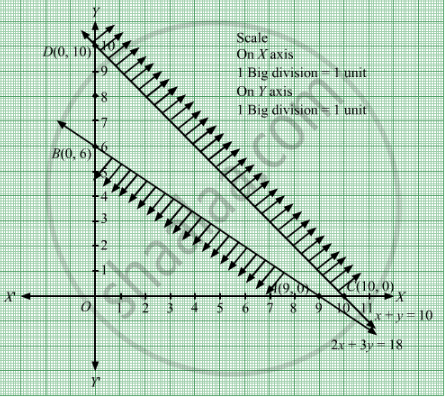# The Maximum Value of Z = 4x + 2y Subjected to the Constraints 2x + 3y ≤ 18, X + Y ≥ 10; X, Y ≥ 0 is (A) 36 (B) 40 (C) 20 (D) None of These - Mathematics

MCQ
Sum

The maximum value of Z = 4x + 2y subjected to the constraints 2x + 3y ≤ 18, x + y ≥ 10 ; xy ≥ 0 is

#### Options

•  36

• 40

•  20

•  none of these

#### Solution

none of these
We need to maximize the function Z = 4x + 2y
Converting the given inequations into equations, we obtain

$2x + 3y = 18, x + y = 10, x = 0\text{ and } y = 0$

Region represented by 2x + 3y ≤ 18 :
The line 2x + 3y = 18 meets the coordinate axes at A(9, 0) and B(0, 6) respectively. By joining these points we obtain the line 2x + 3y = 18.
Clearly (0,0) satisfies the inequation 2x + 3y ≤ 18. So,the region in xy plane which contain the origin represents the solution set of the inequation 2x + 3y ≤ 18.

Region represented by x y ≥ 10:
The line x y = 10 meets the coordinate axes at $C\left( 10, 0 \right)$ and D(0, 10) respectively. By joining these points we obtain the line x y =10.

Clearly (0,0) does not satisfies the inequation x y ≥ 10. So,the region which does not contain the origin represents the solution set of the inequation x y ≥ 10.

Region represented by x ≥ 0 and y ≥ 0:
Since, every point in the first quadrant satisfies these inequations . So, the first quadrant is the region represented by the inequations x ≥ 0 and ≥ 0.We observe that feasible region of the given LPP does not exist.

Concept: Introduction of Linear Programming
Is there an error in this question or solution?

#### APPEARS IN

RD Sharma Class 12 Maths
Chapter 30 Linear programming
MCQ | Q 5 | Page 67